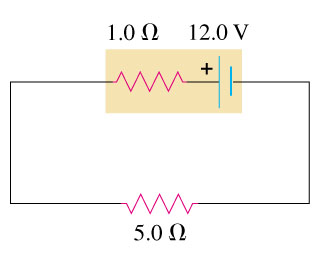# Problem: For the circuit in the figurea) Find the rate of conversion of internal (chemical) energy to electrical energy within the battery.b) Find the rate of dissipation of electrical energy in the battery.c) Find the rate of dissipation of electrical energy in the external resistor.

###### FREE Expert Solution

Current, i:

$\overline{){\mathbf{i}}{\mathbf{=}}\frac{\mathbf{\epsilon }}{\mathbf{R}\mathbf{+}\mathbf{r}}}$

i = 12.0/5.0 + 1.0 = 2.0A

a)

The rate of conversion of internal (chemical) energy to electrical energy within the battery:

83% (295 ratings)###### Problem Details

For the circuit in the figurea) Find the rate of conversion of internal (chemical) energy to electrical energy within the battery.

b) Find the rate of dissipation of electrical energy in the battery.

c) Find the rate of dissipation of electrical energy in the external resistor.